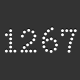Actions

## Bug #4022

closed### Mistake in this cocient of logs followed by %1

Status:
Rejected
Priority:
Normal
Assignee:
-
Target version:
ruby -v:
1.9.1
Backport:
[ruby-core:33039]

Description

=begin
I get a wrong answer when I apply the following expression: Math.log(4913)/Math.log(17) % 1
This happens in ruby 1.9.1 and in ruby 1.8.7
With other set of numbers the answers are correct

irb(main):001:0> Math.log(4913)/Math.log(17) % 1
=> 1.0
irb(main):002:0> Math.log(4913)/Math.log(17)
=> 3.0
irb(main):003:0> 3.0 % 1
=> 0.0
irb(main):004:0> (Math.log(4913)/Math.log(17)) % 1
=> 1.0
=end

Related issues

 Related to Ruby 1.8 - Bug #4023: to_i pathological case in 1.8.7, 1.9.2 Rejected 11/04/2010 Actions Is duplicate of Ruby master - Bug #3746: Incorrect Float subtraction Rejected 08/26/2010 Actions
Actions #1

####Updated by judofyr (Magnus Holm)over 10 years ago

=begin
That's just how floats work:

Math.log(4913)/Math.log(17) == 3.0
=> false
"%.50f" % (Math.log(4913)/Math.log(17))
=> "2.99999999999999955591079014993738383054733276367188"
"%.50f" % 3.0
=> "3.00000000000000000000000000000000000000000000000000"

// Magnus Holm

On Wed, Nov 3, 2010 at 17:52, Daniel Silberschmidt redmine@ruby-lang.orgwrote:

Bug #4022: Mistake in this cocient of logs followed by %1
http://redmine.ruby-lang.org/issues/show/4022

Author: Daniel Silberschmidt
Status: Open, Priority: Normal
Target version: 1.9.1
ruby -v: 1.9.1

I get a wrong answer when I apply the following expression:
Math.log(4913)/Math.log(17) % 1
This happens in ruby 1.9.1 and in ruby 1.8.7
With other set of numbers the answers are correct

irb(main):001:0> Math.log(4913)/Math.log(17) % 1
=> 1.0
irb(main):002:0> Math.log(4913)/Math.log(17)
=> 3.0
irb(main):003:0> 3.0 % 1
=> 0.0
irb(main):004:0> (Math.log(4913)/Math.log(17)) % 1
=> 1.0

http://redmine.ruby-lang.org

That's just how floats work:>> Math.log(4913)/Math.log(17) == 3.0=> false>> "%.50f" % (Math.log(4913)/Math.log(17))=> "2.99999999999999955591079014993738383054733276367188"

>> "%.50f" % 3.0=> "3.00000000000000000000000000000000000000000000000000"// Magnus Holm
On Wed, Nov 3, 2010 at 17:52, Daniel Silberschmidt <redmine@ruby-lang.org> wrote:

Bug #4022: Mistake in this cocient of logs followed by %1
http://redmine.ruby-lang.org/issues/show/4022

Author: Daniel Silberschmidt
Status: Open, Priority: Normal
Target version: 1.9.1
ruby -v: 1.9.1

I get a wrong answer when I apply the following expression: Math.log(4913)/Math.log(17) % 1
This happens in ruby 1.9.1 and in ruby 1.8.7
With other set of numbers the answers are correct

irb(main):001:0> Math.log(4913)/Math.log(17) % 1
=> 1.0
irb(main):002:0> Math.log(4913)/Math.log(17)
=> 3.0
irb(main):003:0> 3.0 % 1
=> 0.0
irb(main):004:0> (Math.log(4913)/Math.log(17)) % 1
=> 1.0

----------------------------------------
http://redmine.ruby-lang.org

=end

Actions #2

####Updated by naruse (Yui NARUSE)over 10 years ago

• Status changed from Open to Rejected
Actions

Also available in: Atom PDF